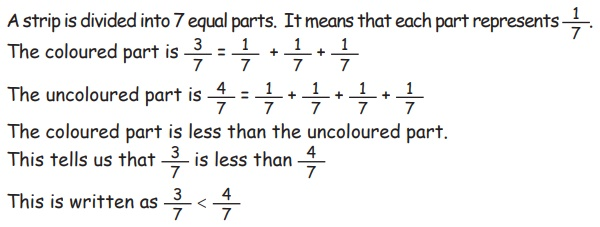Home | | Maths 5th Std | Comparing like fractions

# Comparing like fractions

To compare like fractions, check the numerators of the given fractions. In like fractions, the fraction with the greater numerator is the greater fraction.

Comparing like fractions

To compare like fractions, check the numerators of the given fractions.

Example 6.9SolutionA strip is divided into 7 equal parts. It means that each part represent 1/7

To coloured part is 3/7 = 1/7 + 1/7 +1/7

The uncoloured part is 4 /7 = 1/7 + 1/7 +1/7 +1/7

The coloured part is less than the uncoloured part.

This tells us that 3/7 is less than 4/7

This is written as 3/7 < 4/7

Example 6.10

Compare the different shaded parts in the following figure.Solution

This strip is divided into 5 equal parts. 2 out of 5 parts have one colour and 1 has another colour.

The fraction of blue coloured pard = 2/5

The fraction of yellow coloured part = 1/5

Comparing the numerators 2 and 1, we find 2 > 1

Thereford 2/5 > 1/5In like fractions, the fraction with the greater numerator is the greater fraction.

Tags : Fractions | Term 3 Chapter 6 | 5th Maths , 5th Maths : Term 3 Unit 6 : Fractions
Study Material, Lecturing Notes, Assignment, Reference, Wiki description explanation, brief detail
5th Maths : Term 3 Unit 6 : Fractions : Comparing like fractions | Fractions | Term 3 Chapter 6 | 5th Maths